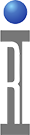Why Measure Phase and Magnitude

1) To Measure Complex Impedance
Resistance with Capacitance and Inductive components
2) Complete Characterization of Linear Networks
3) Transmission Distortion Effects
Deviation from Linear Phase
Bandwidth
4) Vector Accuracy Enhancement
De-imbedding of measurement results
Characterization of Systematic Errors
5) Time Domain Transforms

Before we discuss S Parameters in detail, it may be useful to understand why we need to measure S Parameters of an RF device and why phase information is useful. S Parameter measurements (both amplitude and phase) are used to characterize the transfer characteristics (input to output response) of an RF device and to determine the complex impedance (both resistive and reactive components) of the device’s RF inputs and outputs. S Parameter measurements are also useful for characterizing the device’s transmission errors such as signal distortion thru the device due to nonlinear changes in-phase and group delay. With S Parameter data (both magnitude and phase) you can carefully monitor the impact of small changes in the manufacturing process on the device’s RF performance (in order to improve the manufacturing process) and improve the RF CAD models used to design the devices.

Another benefit of measuring both amplitude and phase is the ability to characterize and mathematically eliminate several system measurement errors. Measurement errors are caused by cable/connection/switch losses, impedance mismatches, changes in coupler directivity over frequency, and source and load mismatch errors. Characterizing and mathematically eliminating these errors significantly improves both amplitude and phase measurement accuracy when compared to scalar (magnitude only) measurement systems. Systems capable of measuring both the magnitude and phase response of a device are referred to as vector network measurement systems or vector network analyzers (VNA's). Systems only capable of measuring amplitude are referred to as scalar measurement systems or scalar network analyzers.

Vector Network Measurement

Reflection Measurements Transmission Measurements
Input VSWR Gain / Loss
Return Loss Isolation
Input Impedance Insertion Phase
Reflection Coefficient Group Delay
S Parameter S11 S Parameters S21, S12

To fully analyze the magnitude and phase characteristics of a typical two port device (DUT with two RF connections), it may require looking at the data in different ways. A vector network analyzer system not only measures the complex reflected or transmitted data but provides you the flexibility to present the data in many different formats. Reflection measurements include Input VSWR, Return Loss, Input Impedance, Reflection Coefficient, and S Parameter S11. Transmission measurements include Gain/Loss, Isolation, Insertion Phase, Reflection Coefficient, Group Delay, and S Parameters S21, S12.

Vector Network Analyzer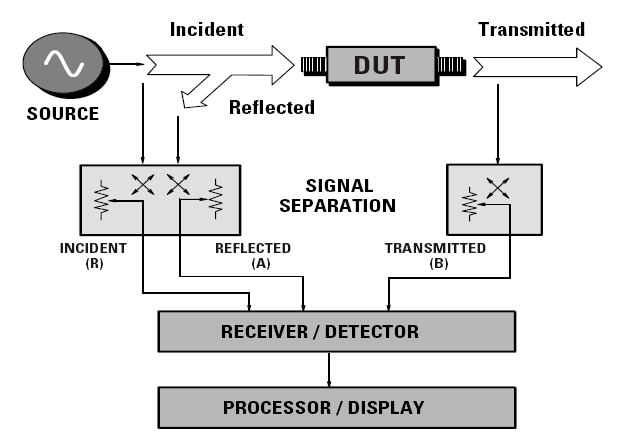A typical vector network analyzer (VNA) system consists of four major parts. The Stimulus Source provides the incident signal. The S Parameter Test Set provides the signal separation of the incident, reflected and transmitted signals. The System Receiver converts the microwave signals to lower intermediate frequency (IF) signals, measures the signals with a complex demodulator (I & Q demodulator) and digitizes the I (in-phase) and Q (quadrature) signal components. Finally, a Signal Processor or System Computer processes the digitized signal data and displays the test results on a viewable screen.

What are these signals that the S parameter Test Set provides to the System Receiver? The incident and transmitted signals are fairly intuitive. The incident signal is applied to the RF input of the Device Under Test (DUT). The signal transmitted through the device to the output of the DUT is the transmitted signal. We can measure and compare the amplitude and phase of the incident signal applied with the transmitted signal received to determine the transmission parameters. For example, the ratio of the transmitted signal's amplitude to the incident signal’s amplitude is the gain or loss through the device.

RF Reflected Signals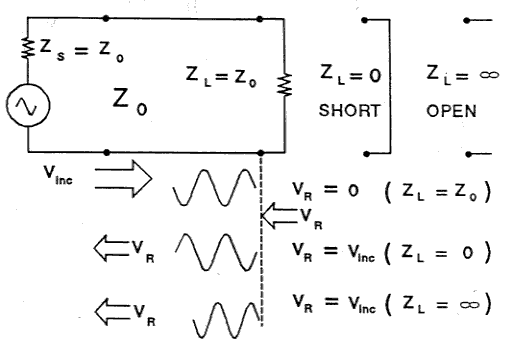The reflected signals are more complex to understand. To help explain what is meant by reflected signals and how they are generated let us look at three transmission line examples. In each example we have an incident signal of RF or microwave energy from a source with an output impedance of 50 ohms. We know that the signal will deliver maximum power to a transmission line if the transmission line’s impedance is the same or equal to the source impedance (i.e. 50 ohm impedance transmission line with infinite length.)

In the first example, we terminate the transmission line with a 50 ohm termination. All of the energy flowing from the source will be absorbed by the termination. This is because the incident signal cannot distinguish between a Z0 load and a Z0 transmission line of infinite length. In the second example, we terminate the transmission line with a short circuit. The short circuit has 0 ohms and can not dissipate any power and the energy is reflected back towards the source. Since the short circuit can not support any voltage (Vshort= 0 volts), the reflected voltage wave must be equal in magnitude and 180° out of phase with the incident voltage wave in order for the voltage sum at the short to be equal to zero. Similarly, in the last example, we terminate the transmission line with an open. The open circuit has infinite impedance and can not support any current and thus can not dissipate any power. The energy is reflected back towards the source in phase and of the same magnitude as the incident wave at the open.

Reflection Coefficient, Return Loss & SWR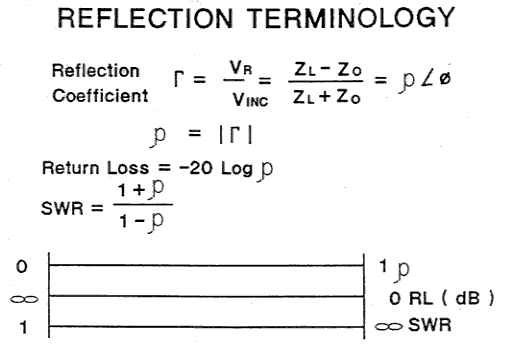We have established that the reflected signal is a function of both the load impedance and the characteristic impedance of the incident signal. The ratio of the reflected voltage to the incident voltage (as shown in the image) is defined as the reflection coefficient, Γ (gamma). The reflection coefficient has both a magnitude and a phase term. The magnitude of the reflection coefficient is defined as ρ (rho) and varies between the values of 0 and 1. Return loss in dB is defined as -20 log ρ. This results in a positive dB number between 0 dB (ρ=1) to infinite dB (ρ=0). Standing Wave Ratio (SWR) is defined as the ratio of the maximum standing wave voltage to the minimum standing wave voltage (Emax/Emin). It is also related to ρ by the equation shown. SWR varies between 1 and (infinity).

Group Delay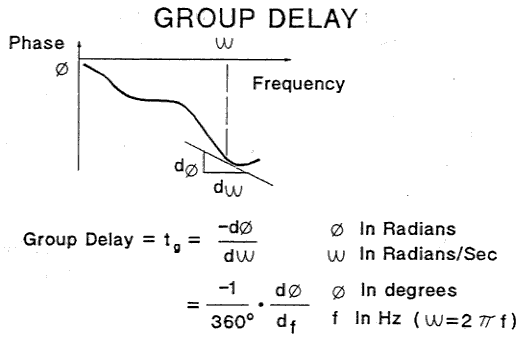Another useful parameter is Group Delay. Group Delay is defined as the negative rate of change in the transmission phase response of a device with respect to the change in the RF frequency. This is equal to the derivative of the phase response with respect to frequency. For example, a device with a linear phase shift versus RF frequency would have a constant rate of change with respect to frequency and therefore a constant group delay. The units for group delay are seconds which indicates that group delay is a measure of transit time through the Device Under Test for a particular frequency.

https://roos.com/docs/RBEH-8EKBBT
ROOS INSTRUMENTS CONFIDENTIAL AND PROPRIETARY